# http www comp nus edu sgcs 2100 Lecture

• Slides: 25http: //www. comp. nus. edu. sg/~cs 2100/ Lecture #14 Logic Circuits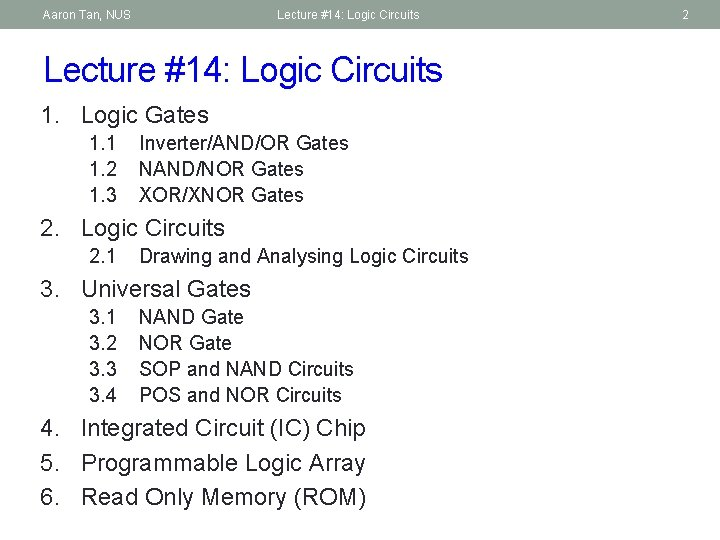Aaron Tan, NUS Lecture #14: Logic Circuits 1. Logic Gates 1. 1 1. 2 1. 3 Inverter/AND/OR Gates NAND/NOR Gates XOR/XNOR Gates 2. Logic Circuits 2. 1 Drawing and Analysing Logic Circuits 3. Universal Gates 3. 1 3. 2 3. 3 3. 4 NAND Gate NOR Gate SOP and NAND Circuits POS and NOR Circuits 4. Integrated Circuit (IC) Chip 5. Programmable Logic Array 6. Read Only Memory (ROM) 2Aaron Tan, NUS Lecture #14: Logic Circuits 3 1. Logic Gates Gate symbols a AND OR NOT NAND NOR EXCLUSIVE OR Symbol set 2 Symbol set 1 b a a b a b a+b a' (a b)' (a+b)' a b (ANSI/IEEE Standard 91 -1984) a & a b b a a b a b 1 a+b 1 a' & (a b)' 1 (a+b)' =1 a b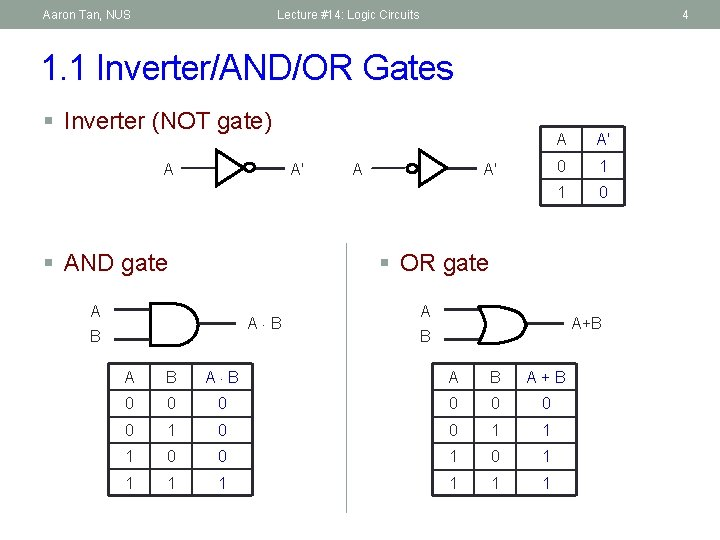Aaron Tan, NUS Lecture #14: Logic Circuits 4 1. 1 Inverter/AND/OR Gates § Inverter (NOT gate) A A' § AND gate A A' 0 1 1 0 § OR gate A A B B A A+B B A B A+B 0 0 0 0 1 1 1 0 0 1 1 1 1Aaron Tan, NUS Lecture #14: Logic Circuits 5 1. 2 NAND/NOR Gates § NAND gate A (A B)' B A B (A B)' 0 0 1 1 1 0 § NOR gate A A B (A + B)' 0 0 1 0 1 0 0 1 1 0 A (A B)' B NAND (A + B)' B Negative-OR A (A + B)' B NOR Negative-AND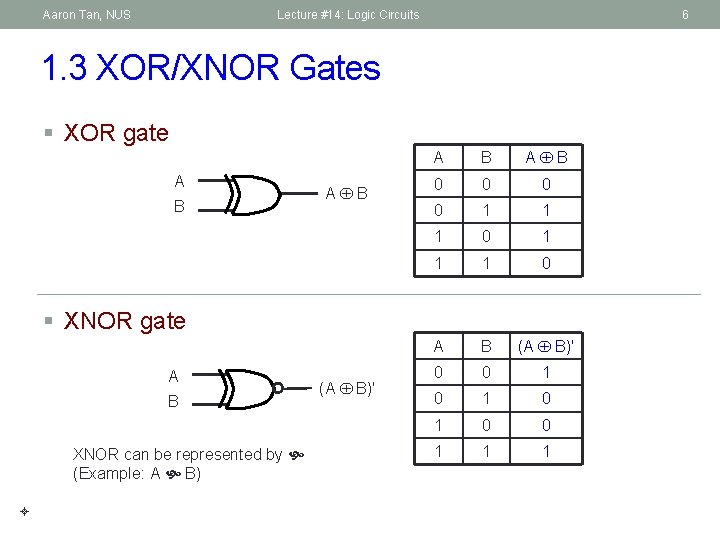Aaron Tan, NUS Lecture #14: Logic Circuits 6 1. 3 XOR/XNOR Gates § XOR gate A B A B 0 0 1 1 1 0 A B (A B)' 0 0 1 0 1 0 0 1 1 1 § XNOR gate A B XNOR can be represented by (Example: A B) (A B)'Aaron Tan, NUS Lecture #14: Logic Circuits 7 2. Logic Circuits (1/2) § Fan-in: the number of inputs of a gate. § Gates may have fan-in more than 2. Every input must be connected in a working circuit! § Example: a 3 -input AND gate § Given a Boolean expression, we may implement it as a logic circuit. § Example: F 1 = x y z' (note the use of a 3 -input AND gate) x y z F 1 z'Aaron Tan, NUS Lecture #14: Logic Circuits 8 2. Logic Circuits (2/2) § Example: F 2 = x + y' z x y' z F 2 x y y' z z If complemented literals are available x' z y' z If complemented literals are not available § Example: F 3 = x y' + x' z x y' F 2 Draw a solid circle to denote that the wires intersect. x y x. y' F 3 x'. z z x'. z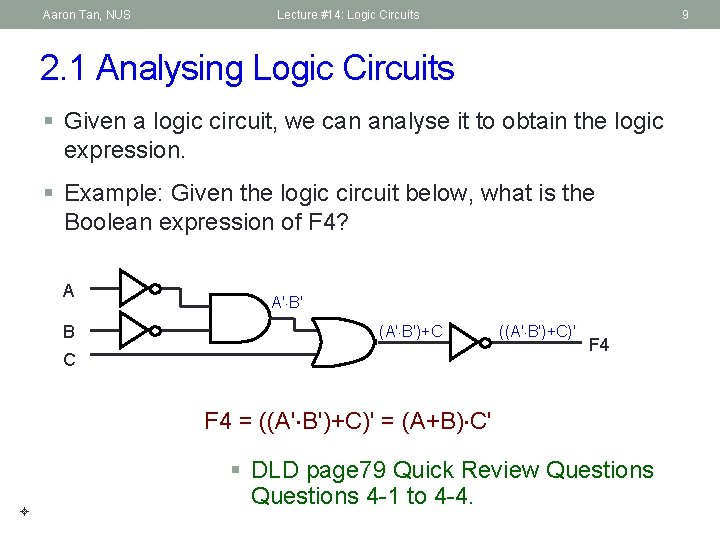Aaron Tan, NUS Lecture #14: Logic Circuits 9 2. 1 Analysing Logic Circuits § Given a logic circuit, we can analyse it to obtain the logic expression. § Example: Given the logic circuit below, what is the Boolean expression of F 4? A B A' B' (A' B')+C C ((A' B')+C)' F 4 = ((A' B')+C)' = (A+B) C' § DLD page 79 Quick Review Questions 4 -1 to 4 -4.Aaron Tan, NUS Lecture #14: Logic Circuits 3. Universal Gates § AND/OR/NOT gates are sufficient for building any Boolean function. § We call the set {AND, OR, NOT} a complete set of logic. § However, other gates are also used: § Usefulness (eg: XOR gate for parity bit generation) § Economical § Self-sufficient (eg: NAND/NOR gates) 10Aaron Tan, NUS Lecture #14: Logic Circuits 3. 1 Universal Gates: NAND Gate § {NAND} is a complete set of logic. § Proof: Implement NOT/AND/OR using only NAND gates. x x y x x' (x∙x)' = x' (idempotency) (x∙y)' x∙y x' x+y y ((x∙y)'∙(x∙y)')' = ((x∙y)')' (idempotency) = x∙y (involution) y' ((x∙x)'∙(y∙y)')' = (x'∙y')' (idempotency) = (x')'+(y')' (De. Morgan) = x+y (involution) 11Aaron Tan, NUS Lecture #14: Logic Circuits 12 3. 2 Universal Gates: NOR Gate § {NOR} is a complete set of logic. § Proof: Implement NOT/AND/OR using only NOR gates. x x y x' (x+x)' = x' (idempotency) x' x∙y ((x+x)'+(y+y)')' = (x'+y')' (idempotency) = (x')'∙(y')' (De. Morgan) = x∙y (involution) x+y ((x+y)'+(x+y)')' = ((x+y)')' (idempotency) = x+y (involution) y' (x+y)' § DLD page 79 Quick Review Questions 4 -6 to 4 -8.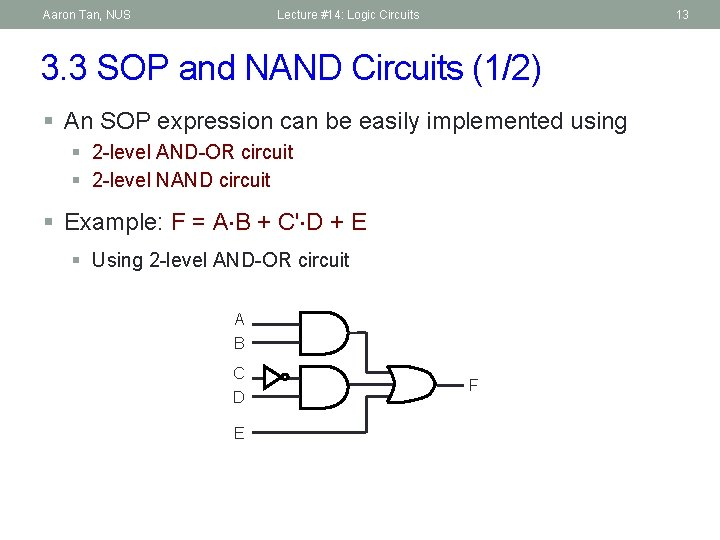Aaron Tan, NUS Lecture #14: Logic Circuits 13 3. 3 SOP and NAND Circuits (1/2) § An SOP expression can be easily implemented using § 2 -level AND-OR circuit § 2 -level NAND circuit § Example: F = A B + C' D + E § Using 2 -level AND-OR circuit A B C D E FAaron Tan, NUS Lecture #14: Logic Circuits 14 3. 3 SOP and NAND Circuits (2/2) § Example: F = A B + C' D + E § Using 2 -level NAND circuit A B C F D E C D F E A B C D E FAaron Tan, NUS Lecture #14: Logic Circuits 15 3. 4 POS and NOR Circuits (1/2) § A POS expression can be easily implemented using § 2 -level OR-AND circuit § 2 -level NOR circuit § Example: G = (A+B) (C'+D) E § Using 2 -level OR-AND circuit A B C D E G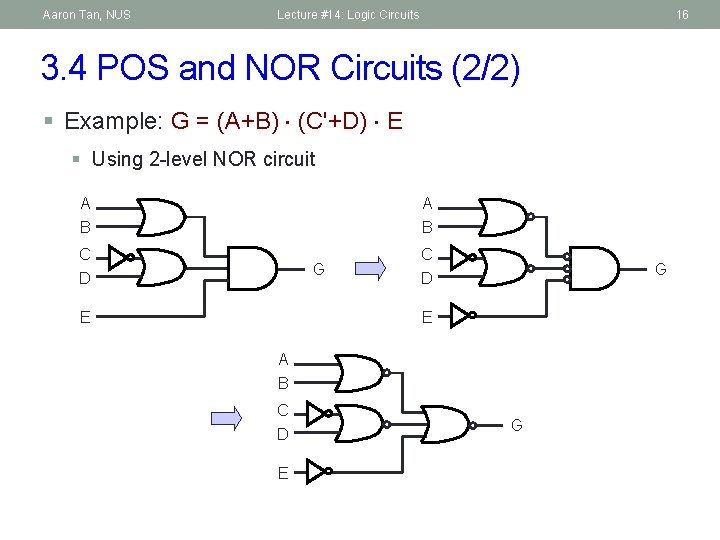Aaron Tan, NUS Lecture #14: Logic Circuits 16 3. 4 POS and NOR Circuits (2/2) § Example: G = (A+B) (C'+D) E § Using 2 -level NOR circuit A B C C G D E A B C D E G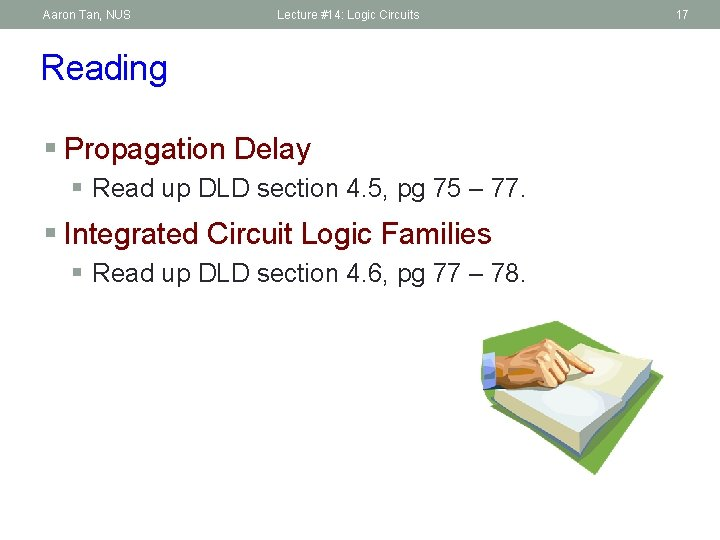Aaron Tan, NUS Lecture #14: Logic Circuits Reading § Propagation Delay § Read up DLD section 4. 5, pg 75 – 77. § Integrated Circuit Logic Families § Read up DLD section 4. 6, pg 77 – 78. 17Aaron Tan, NUS Lecture #14: Logic Circuits 18 4. Integrated Circuit (IC) Chip 14 2 13 3 12 4 11 5 10 6 9 7 8 GND 1 § Example of a 74 LS 00 chip: Quad NAND gates. Vcc = 5 vAaron Tan, NUS Lecture #14: Logic Circuits 19 5. Programming Logic Array (PLA) (1/3) § A programmable integrated circuit – implements sum-of -products circuits (allow multiple outputs). § 2 stages § AND gates = product terms § OR gates = outputs § Connections between inputs and the planes can be ‘burned’. Inputs AND gates Product terms OR gates Outputs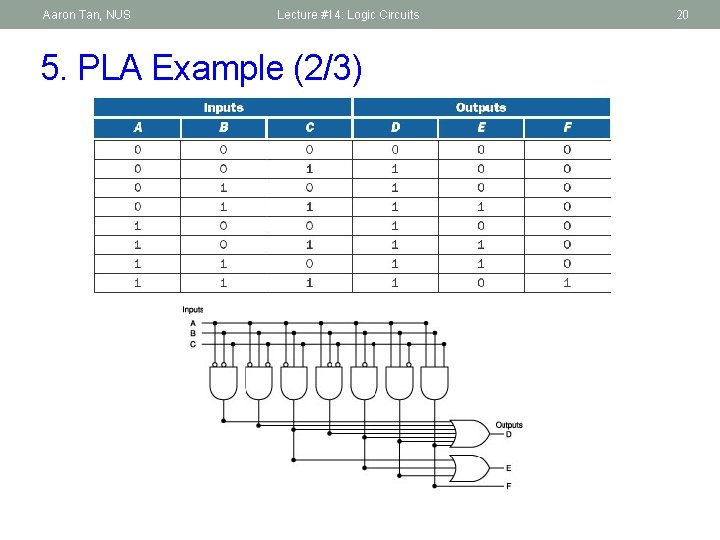Aaron Tan, NUS Lecture #14: Logic Circuits 5. PLA Example (2/3) 20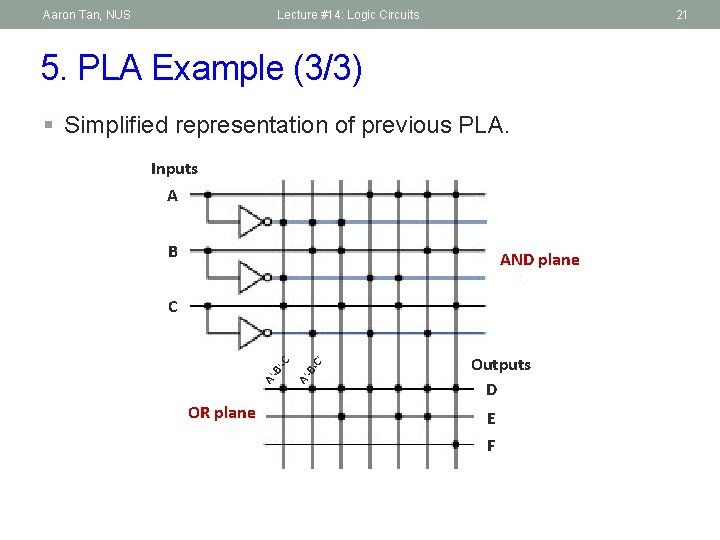Aaron Tan, NUS Lecture #14: Logic Circuits 21 5. PLA Example (3/3) § Simplified representation of previous PLA. Inputs AA BB AND plane OR plane C' A' B ' C CC Outputs D E F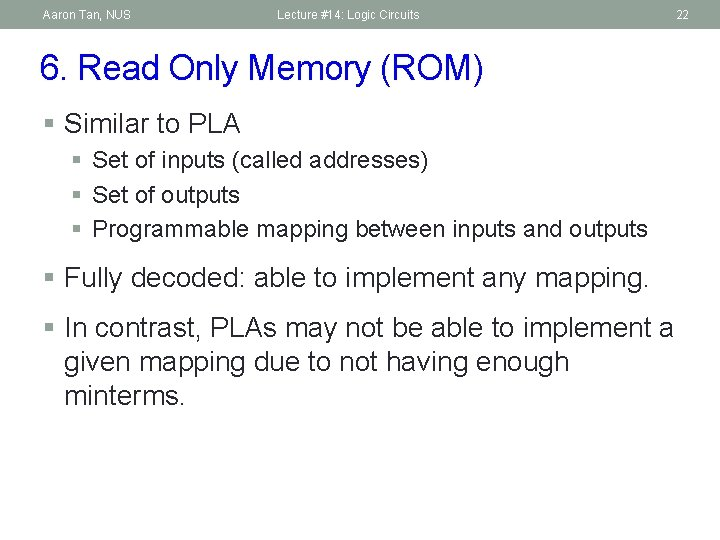Aaron Tan, NUS Lecture #14: Logic Circuits 6. Read Only Memory (ROM) § Similar to PLA § Set of inputs (called addresses) § Set of outputs § Programmable mapping between inputs and outputs § Fully decoded: able to implement any mapping. § In contrast, PLAs may not be able to implement a given mapping due to not having enough minterms. 22Aaron Tan, NUS Lecture #14: Logic Circuits Lab Assignments (1/2) § For the next few labs, you will implement simple circuits using the Logic Trainer 23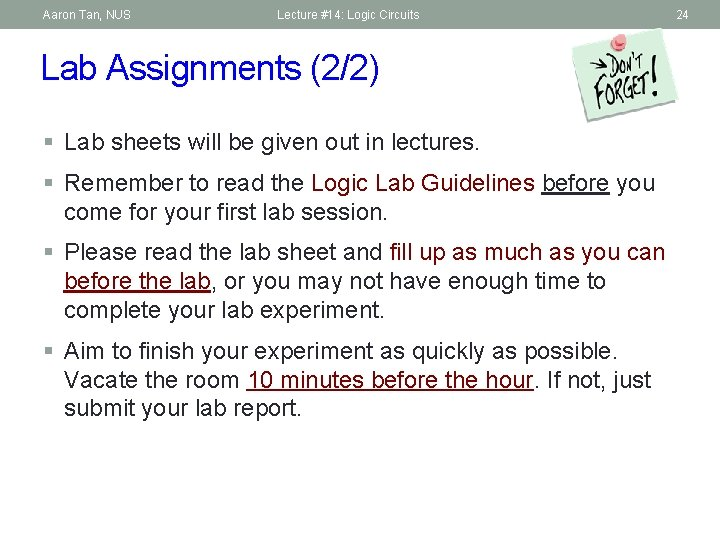Aaron Tan, NUS Lecture #14: Logic Circuits Lab Assignments (2/2) § Lab sheets will be given out in lectures. § Remember to read the Logic Lab Guidelines before you come for your first lab session. § Please read the lab sheet and fill up as much as you can before the lab, or you may not have enough time to complete your lab experiment. § Aim to finish your experiment as quickly as possible. Vacate the room 10 minutes before the hour. If not, just submit your lab report. 24Aaron Tan, NUS Lecture #14: Logic Circuits End of File 25